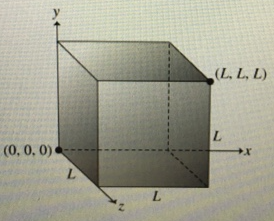# Problem: Flux through a Cube (Figure 1) A cube has one corner at the origin and the opposite corner at the point (L, L, L). The sides of the cube are parallel to the coordinate planes. The electric field in and around the cube is given byE = (a+bx)i + cjPart A. Find the electric flux ϕE through the surface of the cube. Express your answer in terms of a, b, c, and L.Part C. What is the net charge q inside the cube? Express your answer in terms of a, b, c, L, and ε0.

###### FREE Expert Solution

Electric flux,

$\overline{){{\mathbf{\Phi }}}_{{\mathbf{E}}}{\mathbf{=}}\stackrel{\mathbf{⇀}}{\mathbf{E}}{\mathbf{·}}\stackrel{\mathbf{⇀}}{\mathbf{A}}}$

Gauss' law:

$\overline{){{\mathbf{\Phi }}}_{{\mathbf{E}}}{\mathbf{=}}\frac{{\mathbf{q}}_{\mathbf{e}\mathbf{n}\mathbf{c}}}{{\mathbit{ϵ}}_{\mathbf{0}}}}$

(a)

Flux through right side

x = L

E = (a + bL) i + c j

Aright = L2 i

95% (378 ratings)###### Problem Details

Flux through a Cube (Figure 1) A cube has one corner at the origin and the opposite corner at the point (L, L, L). The sides of the cube are parallel to the coordinate planes. The electric field in and around the cube is given by

E = (a+bx)i + cjPart A. Find the electric flux ϕE through the surface of the cube. Express your answer in terms of a, b, c, and L.

Part C. What is the net charge q inside the cube? Express your answer in terms of a, b, c, L, and ε0.